# The Runcinated Tesseract

The runcinated tesseract, also known as the runcinated 16-cell, is a uniform polychoron bounded by 80 cells (32 cubes, 32 triangular prisms, 16 tetrahedra), 208 polygons (64 triangles, 144 squares), 192 edges, and 64 vertices.

It may be constructed by expanding the 8 cubical cells of the tesseract outward radially. The gaps introduced between the cells are filled in by 16 tetrahedra, 32 triangular prisms, and another 24 cubes. Equivalently, it may be constructed by expanding the tetrahedral cells of a 16-cell outward radially, and filling the resulting gaps with 32 cubes and 32 triangular prisms.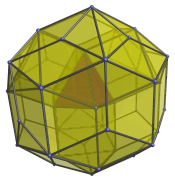It is analogous to the rhombicuboctahedron, a polyhedron which may be constructed by expanding the faces of a cube radially and filling in the gaps with triangles and more squares.

## Structure

We shall explore the structure of the runcinated tesseract by means of its parallel projection into 3D.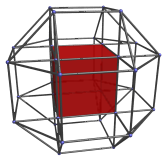The nearest cube to the 4D viewpoint is shown here in red. Surrounding this cubical cell are 6 other cubical cells, as shown in the next image: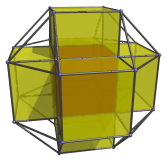These cells appear slightly flattened because they lie at a 45° angle to the 4D viewpoint. In reality, they are perfectly regular cubes. Straddling them are 12 triangular prisms, as shown in the next image: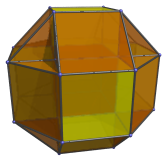The 8 gaps visible in this image, corresponding to the vertices of the central cube. The next image shows these 8 tetrahedra fitted into these gaps: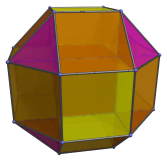The 27 cells we have seen so far lie on the “northern hemisphere” of the runcinated tesseract. Each yellow cube is joined to 6 other cubes at the “equator”: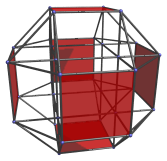These cells appear to be flattened into squares because they are seen at a 90° angle. They are actually perfectly regular cubes.

Overlying the orange triangular prisms shown earlier are 12 more cubical cells that lie on the equator of the runcinated tesseract: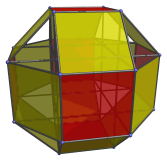These cells also appear flat due to being seen at a 90° angle. They are actually regular cubes.

Finally, there are 8 triangular prisms that lie on the equator of the runcinated tesseract, as shown in the next image: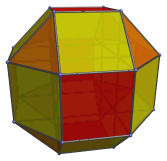These triangular prisms appear flattened into triangles because they are seen at a 90° angle. They join the 8 tetrahedra in the northern hemisphere to 8 other tetrahedra in the southern hemisphere. The cells in the southern hemisphere exactly mirror the layout in the northern hemisphere.

Hence, there are 27 cells in the northern hemisphere, 27 cells in the southern hemisphere, and 26 cells on the equator (6+12 cubes, 8 triangular prisms), making a total of 80 cells.

## Perspective projections

The following image shows the runcinated tesseract in perspective projection. Cells lying on the far side of the polychoron have been culled for clarity.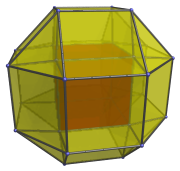The structure of this projection is almost the same as the parallel projection, except that the cubes surrounding the central red cube are foreshortened into frustums instead of cuboids, due to the perspective projection.

The following image shows another perspective projection, this time centered on a tetrahedron:The nearest tetrahedron is shown here in red.

## Coordinates

The coordinates of the vertices of the runcinated tesseract are all permutations of coordinates and changes of sign of:

• (1, 1, 1, 1+√2)

## Geometrical Relationships

The runcinated tesseract can be augmented with 8 cubical pyramids to form a CRF polychoron, the octa-augmented runcinated tesseract, which contains elongated square bipyramid (J15) cells.

Last updated 17 Jun 2019.